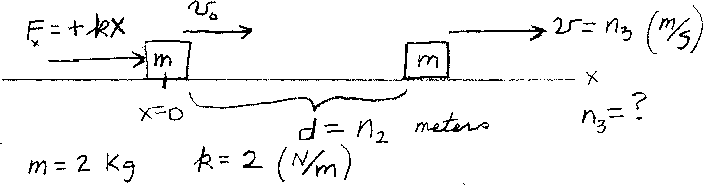Problem C8: An object of mass m=2 Kg is subject to a force on the x-axis of the form Fx = +k x, where k=2 Newtons/meter. When the object is at the origin it's velocity is v0 = n1 meters/sec. What is the objects speed v after it has traveled a distance d = n2 meters on the x-axis. If v = n3 meters/sec, What is n3?n1 = n2 = Input n3:
If you are currently in my class, you can record your grade by entering your name and student ID number (without the leading zeros) below and clicking on "record grade".
 First Name = Last Name = ID = Problem: